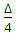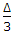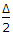# Civil Engineering - Surveying - Discussion

### Discussion :: Surveying - Section 3 (Q.No.45)

45.

If Δ is the angle of deflection of the curve, T1 and T2 are its points of tangencies, the angle between the tangent at T1 and long chord T1 T2 will be

 [A].[B].[C].[D]. Δ [E]. 2 Δ.

Explanation:

No answer description available for this question.

 Gajan Rana said: (Mar 29, 2017) Length of the long chord:- T1T2 = 2R SinΔ/2.

 Meghanada said: (Nov 20, 2017) δ= Angle deflection of the curve. T1 & T2 = points Of Tangecies. Length of the long chod- =T1/T2= 2R Sin δ/2. (Right Answer = δ/2).

 Meghanada said: (Nov 21, 2017) Δ= Angle deflection of the curve. T1 & T2 = points Of Tangecies. Length of the long chod- =T1/T2= 2R Sin Δ /2. (Right Answer = Δ/2).

 Pradeep said: (Jul 6, 2019) Chord length = 2 R sin(δ/2). Tangent length = R tan (δ/2).